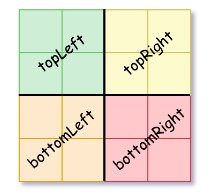New update is available. Click here to update.

Last Updated: 25 Mar, 2021
Difficulty: Moderate

## PROBLEM STATEMENT

#### We can construct a Quad-Tree from a two-dimensional area using the following steps:

``````If the current grid has the same value (i.e., all 1's or all 0's) set isLeaf to true and set 'value' to the value of the grid and set the four children to null and stop.

If the current grid has different values, set “isLeaf” to false and set value to  -1, and divide the current grid into four sub-grids as shown in the image.

Repeat the above steps for each of the children with the proper sub-grid.
``````##### Input Format
``````The first line contains an integer 'T' which denotes the number of test cases or queries to be run. Then the test cases follow.

The 1st line of each test case contains one integer 'N', representing the size of the square matrix.

The next 'N' lines contain 'N' integers separated by spaces describing rows of matrix 'matrix' (each element of ‘matrix’ is either 0 or 1).
``````
##### Output Format
``````The output represents the Quad-Tree in a level order manner, with each node represented as a comma-separated value isLeaf, value.

If the value of “isLeaf” or “value” is 1 we represent it as 1, 1, and if the value of “isLeaf” or “value” is 0 we represent it as 0, 0, and if the “value” is -1 we represent it as -1.
``````
##### Note :
``````You do not need to print anything. It has already been taken care of. Just implement the given function.
``````
##### Constraints:
``````1 <= T <= 10
N = 2^y where 0 <= y <= 10
matrix[i][j] = 0 or 1

Time Limit: 1 second
``````